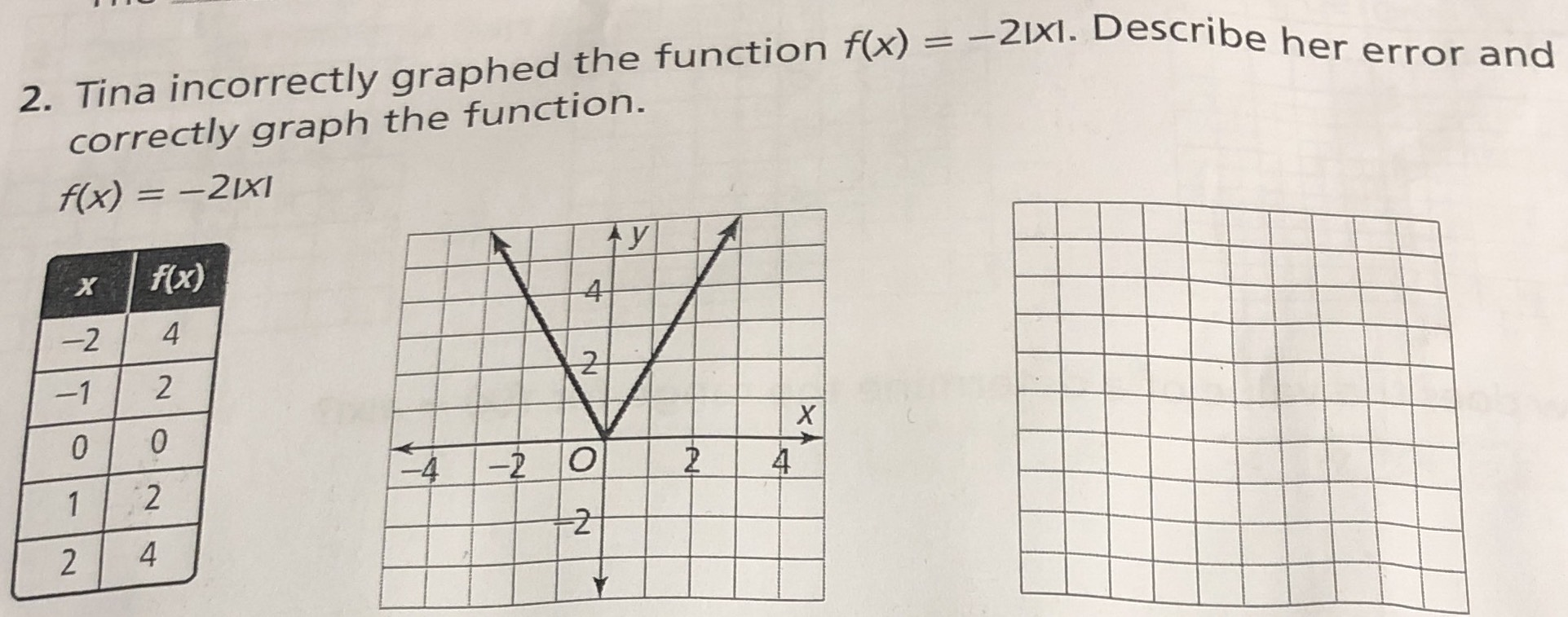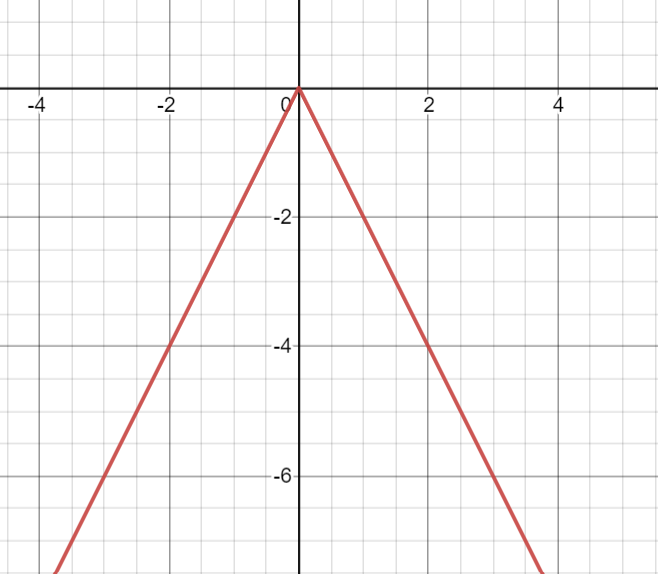### ¿Todavía tienes preguntas de matemáticas?

Pregunte a nuestros tutores expertos
Algebra
PreguntaTina incorrectly graphed the function $$f ( x ) = - 2 | x |$$ . Describe her error and correctly graph the function.

$$f ( x ) = - 2 | x |$$